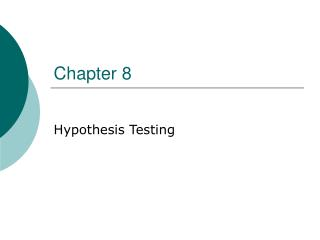DownloadDownload PresentationChapter 8

# Chapter 8

Download Presentation## Chapter 8

- - - - - - - - - - - - - - - - - - - - - - - - - - - E N D - - - - - - - - - - - - - - - - - - - - - - - - - - -
##### Presentation Transcript

1. Chapter 8 Hypothesis Testing

2. 8.2 Null and Alternative Hypothesis Hypothesis testing is the process we go through to decide whether or not the conjecture is true.

3. 8.2 Test Value If the observed (sample) mean is a lot different than the hypothesized population mean, we conclude the hypothesized mean is incorrect.

4. 8.2 Identifying Null and Alternative Hypothesis

5. 8.2 Type I and II Errors

6. 8.2 Type I and II Errors

7. 8.2 Type I and II Errors

8. 8.2 Two Tailed vs. One Tailed Test Bottom pg 394 has English to symbols translations.

9. 8.2 One Tailed Test; α = .01 1 - .01 = .99 Look up .49, what value of z do you get? .5 - .01 = .49 2.33 Critical Value

10. 8.2 One Tailed Test; α = .01 Right-Tail Test Left-Tail Test

11. 8.2 Two Tail Test If α = .01

12. 8.2 Critical Values α= .10,.05,.01

13. 8.2 Example 1

14. 8.2 Example 1

15. 8.2 Example 1 .4901 2.33

16. 8.3 z Test for a Mean

17. 8.3 Steps for Hypothesis Testing

18. 8.3 Example 2

19. 8.3 Example 2

20. 8.3 Example 3

21. 8.3 Example 3

22. 8.3 P-value Method for Hypothesis Testing

23. 8.3 Example 4

24. 8.3 Example 4

25. 8.3 Example 5

26. 8.3 Example 5

27. 8.3 Decision Rules for P-Values If you are not given an α value: X X ? √ 0 .05 .10 .01

28. 8.4 t Test for a Mean In chapter 7, when n <30 and σ was unknown we used tα/2 values verses z α/2 values for our confidence intervals.

29. 8.4 Example 6

30. 8.4 Example 7 Look at the One tail α heading Use the .01 column Go down to row 22 -2.508 Look at the Two tails α heading Use the .10 column Go down to row 18 -1.734 and +1.734

31. 8.4 Example 8

32. 8.4 Example 8

33. 8.4 Example 9 d.f. = 11 – 1 = 10 Look in row 10 for t-value 2.056 (or the closest two values you can find. For the t-value up to the top of the column; use the One-tail heading.

34. 8.4 Example 9

35. 8.4 Example 9 .025 < p-value < .05 If we were testing at the α = .05 level would we accept or reject H0? Reject because p-value < .05

36. 8.4 Example 10 Look in row 5 for t-value 2.983 Use the Two-tails heading .05 .02 2.571 2.983 3.365 .02 < p-value < .05 If α = .01 level would we accept or reject H0? Do not reject since p-value > .02 > .01

37. 8.4 Example 10

38. 8.4 Example 10

39. 8.5 z-Test for Proportion

40. 8.5 Example 11

41. 8.5 Example 11

42. 8.5 Example 11

43. 8.5 Example 11

44. 8.5 Example 11

45. 8.5 Example 11

46. 8.5 Example 12

47. 8.5 Example 12

48. 8.6 X2 Test for σ2 or σ • The areas for Chi-square distribution Table G pg 772 • Left, right and two tailed test • Chi-square distribution different than z and t in that the values are never negative

49. 8.6 Example 13

50. 8.6 Example 13